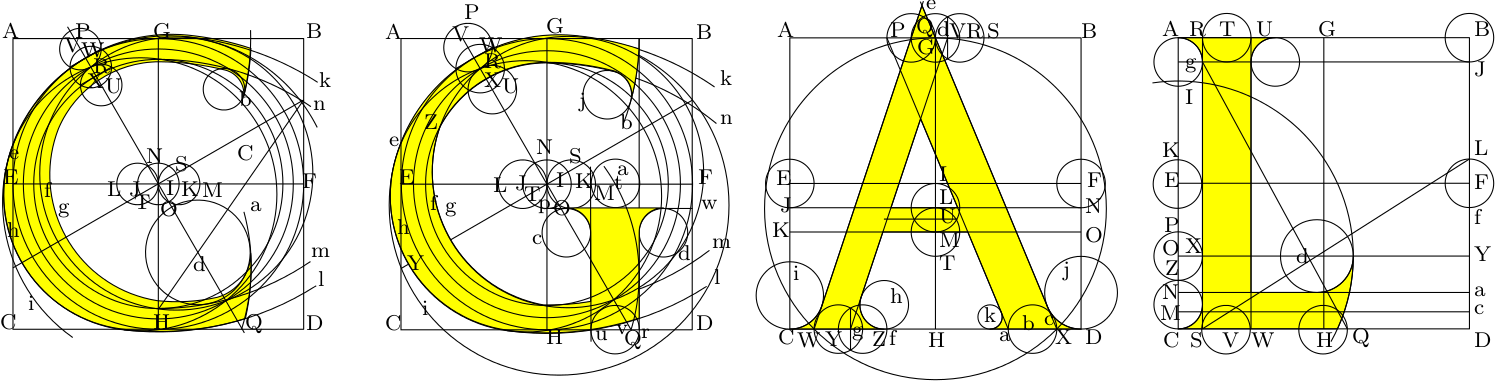# About the Original Logo Design

The letters of the original CGAL logo were made following: David Lance Goines, A Constructed Roman Alphabet: a Geometric Analysis of the Greek and Roman Capitals and of the Arabic Numerals. David R. Gordine, Boston, 1982.Here are, for example, the precise instructions to construct the letter 'C':
• Construct first a square ABCD, and bisect AC at E, BD at F, AB at G, and CD at H.
• Draw the straight lines EF and GH, thereby establishing a point I at their intersection.
• From the center I, describe a circle JIK on EF, the diameter of which is one-ninth the distance AB.
• Using that same radius, describe a circle LJI, thereby establishing a point N on the perimeter of the circle I.
• Using that same radius, describe a circle IKM, thereby establishing a point O on the perimeter of the circle I.
• Draw the straight line NO, and produce it to intersect AG at P and HD at Q.
• From the point I, construct a line at right angles to PQ, thereby establishing on the perimeter of the circle I the points T and S.
• Produce IT to intersect EC at Y.
• From the center I, describe a circle Z tangential to AB, thereby establishing on PN a point R.
• Using the radius IK, describe from the center R a circle VRU.
• Using that same radius, decribe circles from the centers V and U.
• Bisect the intersections of the circles V and R, thereby establishing a point W on VR.
• Bisect the intersections of the circles R and U, thereby establishing a point X on RU.
• From the center I, describe a circle e intersecting the point W.
• From the center I, describe a circle f intersecting the point X.
• From the center S, describe a circle g intersecting the point R.
• Using the same radius, describe a circle h from the center T.
• From the center O describe a circle i tangential to the circles e and h.
• From the center H, describe an arc k tangential to the circle i, and intersecting BF.
• Describe an arc l from the center G tangential to the circle h and intersecting FD.
• From the center G, describe an arc m tangential to the circle f and intersecting FD.
• Describe from the center H an arc n tangential to the circle f and intersecting BF.
• Draw the straight line HF, thereby establishing a point d on IQ.
• From the center Y, describe an arc a intersecting the point Q and the line dF.
• Using the same radius, describe from the center A an arc b intersecting GB and the circle h.
• Using the radius IK, describe a circle c tangential to the interiors of the arcs n and b.
• From the center d, describe a circle tangential to the arc a and to the arc m.
Q.E.F.